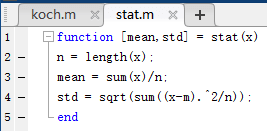# 函数定义

``````function [ret1, re2, ...] = fun_name(in1, in2, ...)
% function body
end
``````

其中方括号里面的ret1等，是最后返回的变量，而圆括号里面的in1等，是调用函数时需要输入的变量，当然也可以没有输入或者输出，保存时，文件名应与函数名保持一致。调用时类似于MATLAB的其他函数，比如qr分解，其中输入与输出的名字可以更改：

``````[matQ, matR] = qr(A)
``````

还可以使用nargin与nargout来判断输入的变量个数与输出的变量个数,具体可以参照帮助文档，比如：

``````function [mean,std] = stat(x)
n = length(x);
m = sum(x) / n;
if nargout == 1
mean = m;
elseif margout == 2
mean = m;
std = sqrt(sum((x-mean).^2/n));
end
end
``````

如果要忽略其中几个参数，可以使用~

``````[~, s] = stat(rand(3))

s =

0.1980    0.2786    0.3550
``````